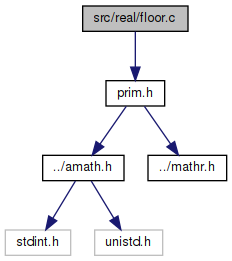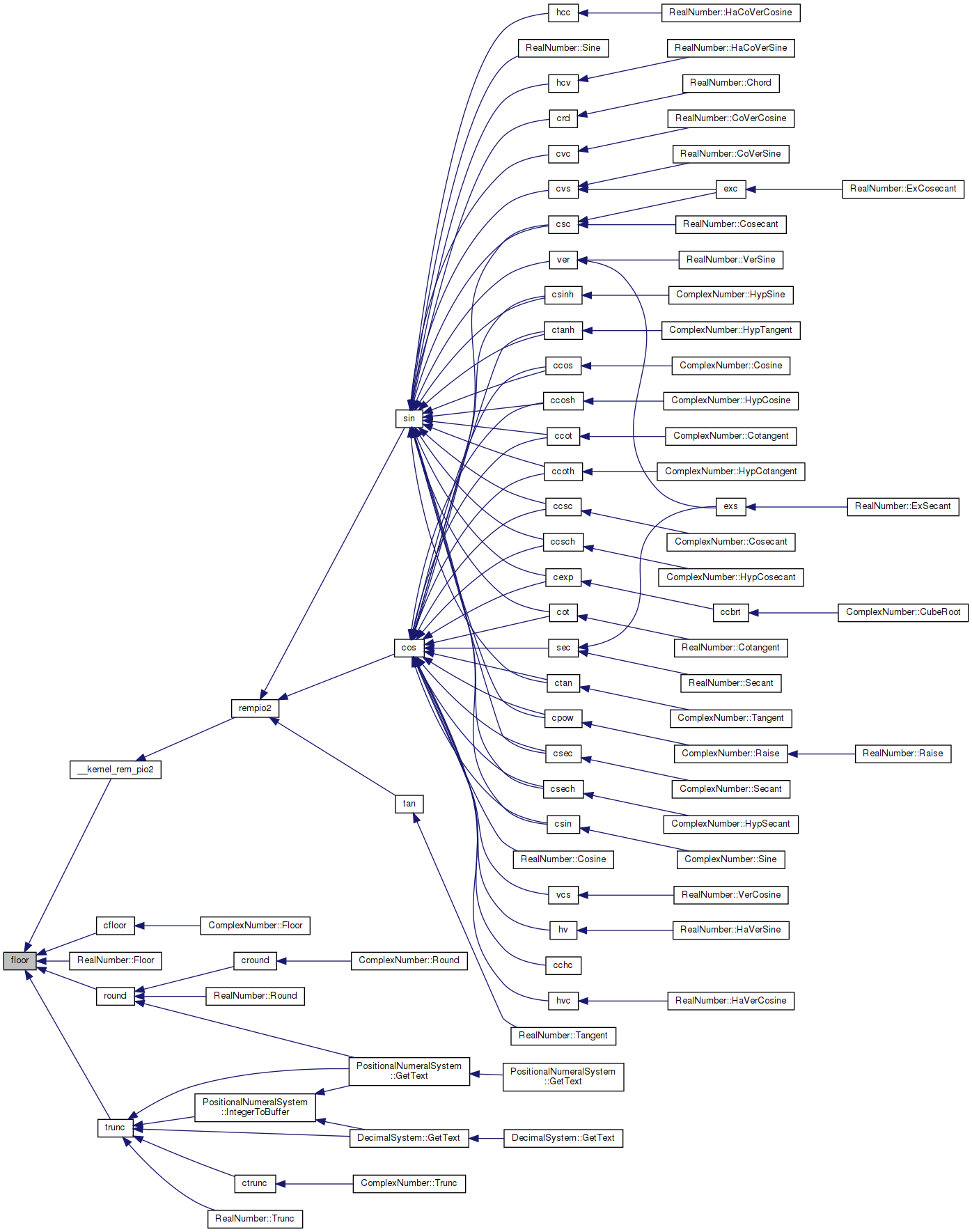amath  1.8.5 Simple command line calculator
floor.c File Reference

Floor function. More...

`#include "prim.h"`
Include dependency graph for floor.c:Go to the source code of this file.

## Functions

double floor (double x)
Floor function. More...

## Variables

static const double huge = 1.0e300

## Detailed Description

Floor function.

Definition in file floor.c.

## ◆ floor()

 double floor ( double x )

Floor function.

Returns
x rounded toward -inf to integral value
```Method:
Bit twiddling```
```Exception:
Inexact flag raised if x not equal to floor(x)
```

Definition at line 62 of file floor.c.

References huge.

Referenced by __kernel_rem_pio2(), cfloor(), RealNumber::Floor(), round(), and trunc().

63 {
64  int32_t i0, i1, j0;
65  uint32_t i, j;
66  EXTRACT_WORDS(i0, i1, x);
67  j0 = ((i0 >> 20) & 0x7ff) - 0x3ff;
68  if (j0 < 20)
69  {
70  if (j0 < 0)
71  { /* raise inexact if x != 0 */
72  if (huge + x > 0.0)
73  { /* return 0*sign(x) if |x|<1 */
74  if (i0 >= 0)
75  {
76  i0 = i1 = 0;
77  }
78  else if (((i0 & 0x7fffffff) | i1) != 0)
79  {
80  i0 = 0xbff00000;
81  i1 = 0;
82  }
83  }
84  }
85  else
86  {
87  i = (0x000fffff) >> j0;
88  if (((i0 & i) | i1) == 0)
89  return x; /* x is integral */
90  if (huge + x > 0.0)
91  { /* raise inexact flag */
92  if (i0 < 0)
93  i0 += (0x00100000) >> j0;
94  i0 &= (~i);
95  i1 = 0;
96  }
97  }
98  }
99  else if (j0 > 51)
100  {
101  if (j0 == 0x400)
102  return x + x; /* inf or NaN */
103  else
104  return x; /* x is integral */
105  }
106  else
107  {
108  i = ((uint32_t)(0xffffffff)) >> (j0 - 20);
109  if ((i1 & i) == 0)
110  return x; /* x is integral */
111  if (huge + x > 0.0)
112  { /* raise inexact flag */
113  if (i0 < 0)
114  {
115  if (j0 == 20)
116  i0 += 1;
117  else
118  {
119  j = i1 + (1 << (52 - j0));
120  if (j < (uint32_t)i1)
121  i0 += 1; /* got a carry */
122  i1 = j;
123  }
124  }
125  i1 &= (~i);
126  }
127  }
128  INSERT_WORDS(x, i0, i1);
129  return x;
130 }
#define INSERT_WORDS(d, ix0, ix1)
Set a double from two 32 bit ints.
Definition: prim.h:187
static const double huge
Definition: floor.c:48
#define EXTRACT_WORDS(ix0, ix1, d)
Get two 32 bit ints from a double.
Definition: prim.h:156
Here is the caller graph for this function:## ◆ huge

 const double huge = 1.0e300
static

Definition at line 48 of file floor.c.

Referenced by floor().